painting 3D Lissajous figuresThe lissajous3d program allows the plotting of 3 dimensional Lissajous graphics.
Below is a reduced picture of this (Windows) program at work: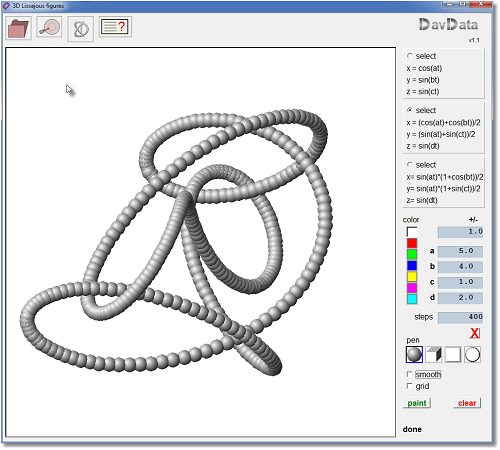Program features

- choice of 3 sets of Lissajous formulas
- choice of 4 pen styles:
- sphere
- cube
- square
- circle
- choice out of 7 colors
- single dots or connected (smooth) lines
- step count from 100..1000
- save settings to disc (*.l3d extension attached)
- save picture (*.bmp)
For the constants a,b,c,d :
- a left mouseclick adds the (+/-) value
- a right click subtracts the (+/-) value.
The (+/-) value itself may be changed also by left- or right mouseclicks.

Interim variable t counts from 0 to the selected stepcount.
Stepcount is selectable between 100 and 1000 in steps of 50.

The menu and buttons are self-explanetory.

Below is pictured a "dotted" and the same "smooth" function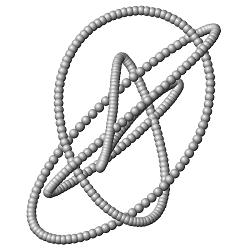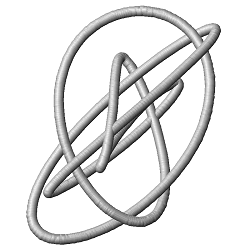single dots smooth

What are Lissajous functions?

The commonly used functions have the format
y = ... x ..., where ... stands for operators and constants.
Plotting such a function in a rectangular coordinate system results in a smooth line.
For a given value of x, only one value of y may result.
So, the painting of circles or ellipses is not possible.

This may be overcome by using so called parametric functions, which have the format
x = ...t...
y = ...t...
Now, both x and y are a function of interim variable t.

Lissajous functions have the form
x = cos(...t)
y = sin(...t)
So, x and y are both periodic functions.
Note: sin and cos are interchangeble.

A circle with radius 5 and center at (0,0) is represented by
x = 5cos(t)
y = 5sin(t)
Angles are measured in degrees, internal calculations are done in radians where 2p radians = 360 degrees.
360 degrees make a full period.
So, in the picture below, t has to increment from 0 to 360 to paint the complete circle.More "interesting" pictures may be obtained by adding constants to the functions or use even
addition or multiplication of periodic functions.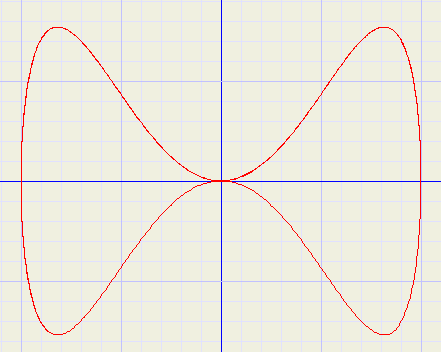x = 2cos(t)y = sin(t) + sin(3t)

Above picture was made with graphics explorer a functions and equations grapher program.

Lissajous 3D

For 3D, a z axis is added to the X and Y axis.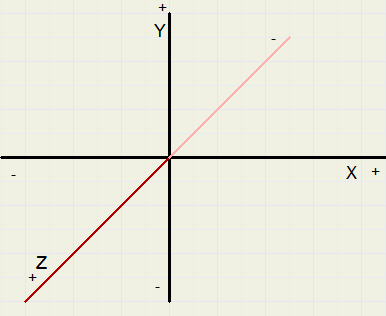3D Lissajous functions have the general form
x = cos(..t)...
y = sin(..t)...
z = sin(..t)...
Note: sin and cos functions are interchangeble.
Click on the download (lightning) button at the top of this page to load the Lissajous-3D program.